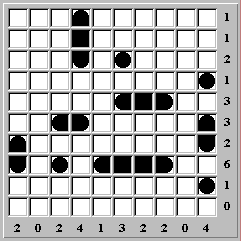World of Puzzles (November 1999)

Analyses of Puzzles

Analysis of Ensign Puzzle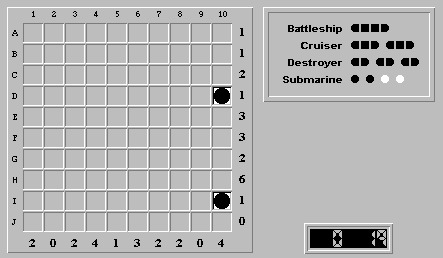Here's the starting board. Finalizing any obvious water square (e.g. (C,9)), we get: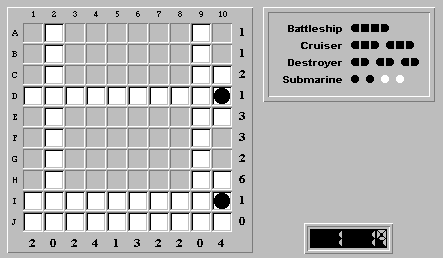In row H, neither (H,3) nor (H,8) can be water, as this would create a five-segment ship along row H. Setting (H,3) and (H,8) to a ship segment gives: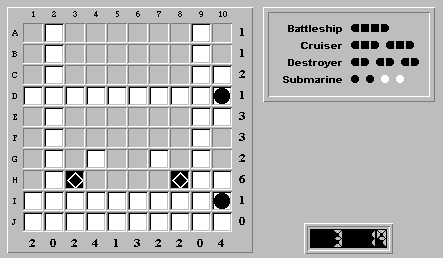There are only two places to position the battleship: (H,3)-(H,6) and (H,5)-(H,8). In either case, the overlapping squares (H,5) and (H,6) are ship segments: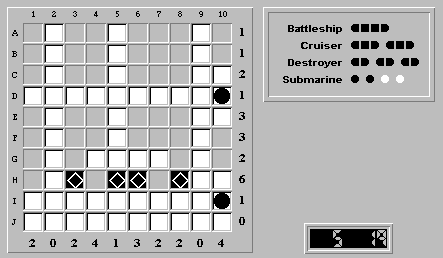There are three possible positions to place the two cruisers: (A,4)-(C,4), (E,6)-(E,8), and (F,6)-(F,8). There are exactly two ways to place both cruisers on the board at the same time. In both ways, a cruiser is placed at (A,4)-(A,6). Placing a cruiser there gives us: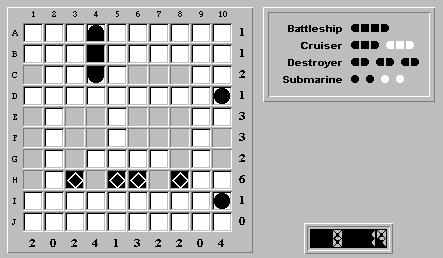Beginning with the fact that the remaining squares in column 10 must be ship segments, the rest of the solution is obvious.